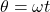## The take-up reel of a cassette tape has a radius of 2.5 cm. Find the length of the tape that passes around the reel in 7.1 s when the reel r

Question

The take-up reel of a cassette tape has a radius of 2.5 cm. Find the length of the tape that passes around the reel in 7.1 s when the reel rotates at an average angular speed of 1.9 rad/s.

in progress 0
2 months 2021-07-29T05:21:23+00:00 1 Answers 2 views 0

s = 0.337 m

Explanation:

First, we will find the angular displacement of the reel:where,

θ = angular displacement = ?

ω = angular speed = 1.9 rad/s

t = time taken = 7.1 s

Therefore,

Now, we will find out the length of tape:

s = rθ

where,

s = length of tape = ?

r = radius of reel = 2.5 cm = 0.025 m

Therefore,